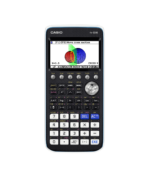Functions Archives - Casio Calculators

# Functions

Displaying Resources

FilterHow to Video
fx-CG50 A-Level GCSE IB
Quick Skill: Calculations Using Coordinate Values

How to extract coordinate values from points of interest in Graph to perform ope...How to Video
fx-CG50 A-Level GCSE IB
Quick Skill: Graphing – Storing and Recalling Functions

How to use Graph Memory to store functions and settings for later recall. You wi...How to Video
fx-CG50 A-Level GCSE IB
Quick Skill: Table – Extracting Values

How to extract either a single column or a complete set of table values. You wil...How to Video
fx-CG50 A-Level GCSE IB
Quick Skill: Graphing – Drawing a Graph and Table

How to draw the graph of a function and create a table of values from the graph....How to Video
fx-CG50 A-Level GCSE IB
Quick Skill: Graphing – Drawing 2 Graphs

How to draw adjacent graphs for comparing functions. You will need to know how t...How to Video
fx-CG50 A-Level GCSE IB
Quick Skill: Graphs – Quick Scale Settings

How to use Zoom to automatically fill the graph with the sketched function, zoom...How to Video
fx-CG50 A-Level IB
Quick Skill: Graphing – Inverse Trigonometric Functions

How to graph the inverse trigonometric functions. Restricting the domain ensures...How to Video
fx-CG50 A-Level IB
Quick Skill: Graphing – Restricting the Domain of a Function

How to restrict the domain of a function when graphing. You will need to know ho...How to Video
fx-CG50 A-Level IB
Quick Skill: Calculations Using Function Notation

How to use function notation to perform operations in the Run-Matrix app. You wi...How to Video
GCSE
Absolute Values fx-83/85GTX

How to use the absolute or modulus function to create a table of values for a fu...How to Video
A-Level GCSE
Table of Values fx-991EX

How to create a table of values of a function with incremental steps. Extend th...How to Video
fx-CG50 A-Level IB
Transforming Functions

How to transform graphs of functions using variable coefficients. Includes trans...How to Video
fx-CG50 A-Level GCSE IB
Table – Calculating Values

How to calculate a table of values for a function. Change the step interval and ...How to Video
fx-CG50 A-Level GCSE IB

How to use Equation to numerically solve a polynomial equation with real roots....How to Video
fx-CG50 A-Level IB
Modulus Function – Graphs and Equations

How to plot the graph of a modulus function. Solve modulus equations both graphi...How to Video
fx-CG50 A-Level GCSE IB
Graphs – Key Points and Intersections

How to find the coordinates of key points such as intercepts and stationary poin...How to Video
fx-CG50 A-Level GCSE IB
Graphs – Getting Started

How to plot the graph of a function, adjust the axes of a graph, move around the...How to Video
fx-CG50 A-Level IB
Graphing – Variable Coefficient Functions

How to explore the effect of using Modify to change the coefficients of a functi...How to Video
fx-CG50 A-Level IB
Graphing – Types of Function

Plot graphs of parametric functions and where x = constant. Plot inequalities an...